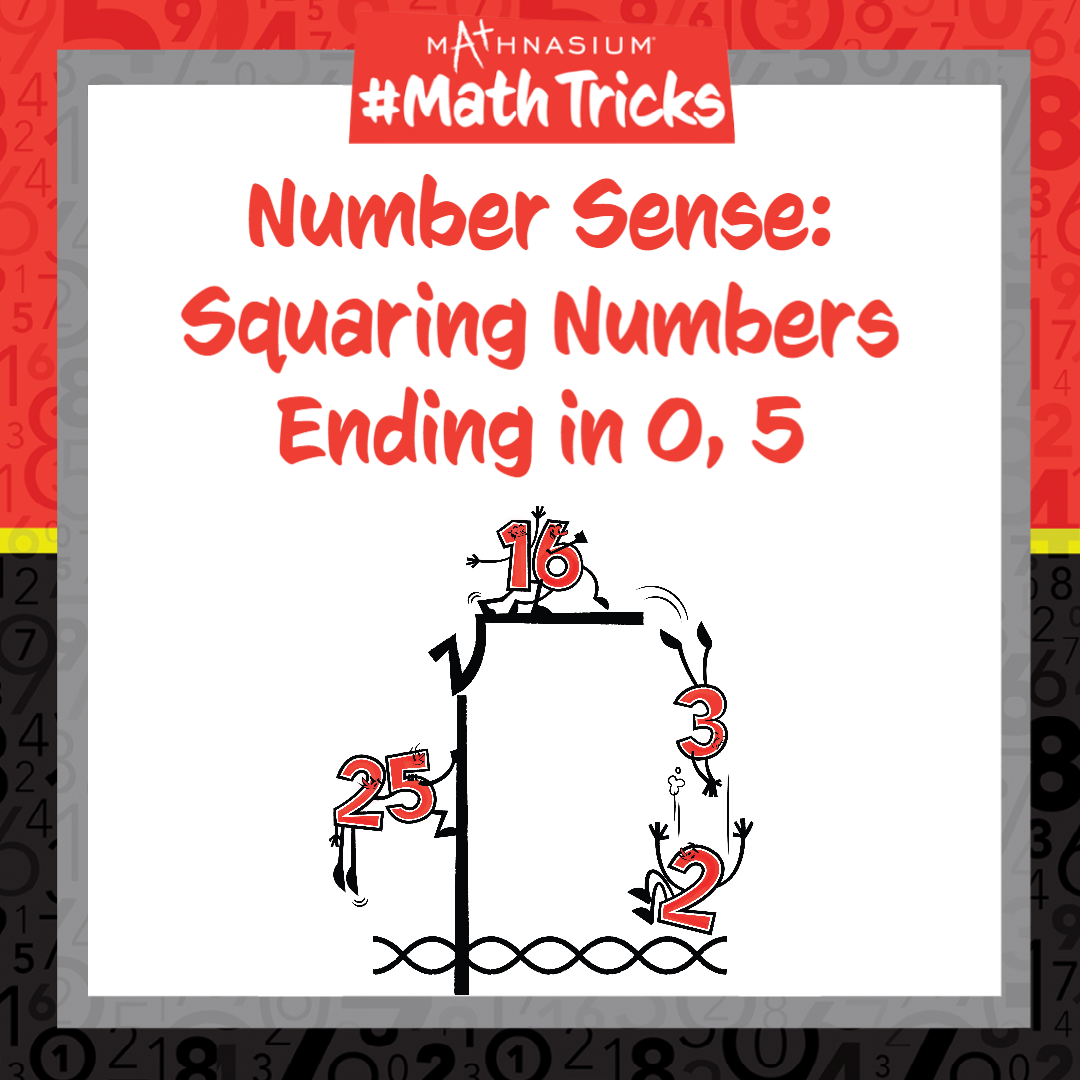877-601-6284
Get Started by Finding a Local Center

# Mathnasium #MathTricks: Number Sense (Squaring Numbers Part 1)

Aug 31, 2022 |Welcome to Mathnasium’s Math Tricks series. Today we are squaring numbers ending in 0 or 5 by observing patterns and applying our number sense.

To square a number, we multiply it by itself. Sometimes, this multiplication might be difficult to compute, but let’s observe a few patterns that might help us out — see if you and your child can spot them!

902 = 8,100
1102 = 12,100
452 = 2,025
1152 = 13,225

Did you notice that the square of a number ending in 0, such as 90, ends in 00? How about that the beginning of the final answer is the square of the digits in front of 0 in the original number? In the case of 90, 92 = 81.

Did you also notice that the square of a number ending in 5, such as 45, ends in 25? And that the beginning of the final answer is equal to the digits in front of the 5 multiplied by those same digits increased by 1? The square of 45 begins with the digits 20 because 4 multiplied by (4+1) is equal to 4×5 = 20. And this happens all the time!

Follow the examples below to observe more patterns and use them to find the square of each number.

##### Example 1: Find 1202.

Step 1: Identify the number-sense pattern for squaring numbers.
Since 120 ends with a 0, we turn to the first number-sense trick.

Step 2: Write down two 0s for the end of the final answer.
_____ 00.

Step 3: Square the digits before the 0.
We square the digits 12: 122 = 12 x 12 = 144.

Step 4: Put the answer to Step 3 in front of the 00 in the final answer.
144 00.

Answer: 120 squared is equal to 14,400.

##### Example 2: Find 1252.

Step 1: Identify the number-sense pattern for squaring numbers.
Since 125 ends with a 5, we turn to the second number-sense trick.

Step 2: Write down 25 for the end of the final answer.
_____ 25.

Step 3: Increase the digits in front of the 5 by 1.
We increase the digits 12 by 1: 12 + 1 = 13.

Step 4: Multiply the original digits in front of the 5 by the digits from Step 3.
We multiply 12 x 13 = 156.

Step 5: Put the answer to Step 4 in front of the 25 in the final answer.
156 25.

Answer: 125 squared is equal to 15,625.

Now, with this strategy, you are ready to square numbers ending in 0 or 5 using the mental math and number sense. Click here for more practice problems, then check your answers here.

If you missed this, or any of our other Math Tricks videos, check them out on our YouTube channel!

## SEE HOW MATHNASIUM WORKS FOR YOUR SITUATION

### My Child is:## OUR METHOD WORKS

Mathnasium meets your child where they are and helps them with the customized program they need, for any level of mathematics.Early LearnersElementary SchoolMiddle SchoolHigh School